Verifying a Trigonometric Equation Identity

Lesson Transcript
Instructor: Yuanxin (Amy) Yang Alcocer

Amy has a master's degree in secondary education and has been teaching math for over 9 years. Amy has worked with students at all levels from those with special needs to those that are gifted.

Trigonometric equation identity, or whether an equation that is true, can be determined or verified by utilizing specific trig identities. Learn some basic identities, and how they apply in provided examples. Updated: 10/20/2021

A Trigonometric Equation Identity

A trigonometric equation identity is a trigonometric equation that is held to be true. Think of these as your regular equations. They have an equals sign and are assumed to be true. Think about what it means to be an equation. Doesn't it mean that the two sides are always equal? Yes, the left side must always equal the right side. For our trigonometric equation identities, instead of numbers or variables, we will have our trigonometric functions of sine, cosine, tangent, cosecant, secant, and cotangent. So, instead of seeing 3 = 1 + 2, we will see cotangent = 1/tangent.

This video lesson is about proving or verifying these identities. How do we do that? We do this by working on just one side of the equation at a time. We work on one side of the equation and work to change it so that it looks like the other side. How do we do that? We do that by using our basic trig identities that we know for sure are true and substituting them in whenever possible.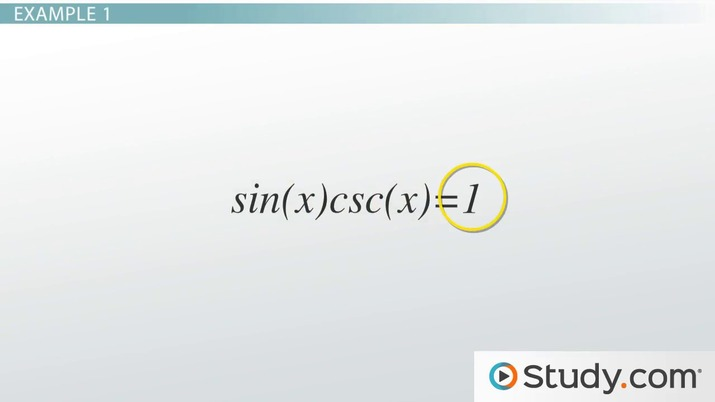An error occurred trying to load this video.

Try refreshing the page, or contact customer support.

Coming up next: Applying the Sum & Difference Identities

You're on a roll. Keep up the good work!

Replay
Your next lesson will play in 10 seconds
• 0:01 A Trigonometric…
• 1:31 Example 1
• 2:53 Example 2
• 3:46 Lesson Summary
Save Save

Want to watch this again later?

Timeline
Autoplay
Autoplay
Speed Speed

Our Basic Identities

What are some basic trig identities that we can use to help us prove our trigonometric equation identities? These include our reciprocal identities of our trig functions.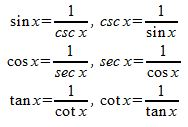We also have our quotient identities.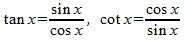It also includes our Pythagorean identities as well as our even-odd identities.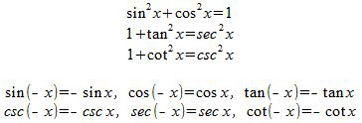There are other identities out there, as well. Unfortunately, there isn't room in this lesson to list them all for you. If you haven't already memorized these proven trig identities, then now is your chance to get started. You will see how easy it will make your problem-solving life.

Example 1

Let's take a look at a couple of examples to see how we can use our basic identities to help us prove other trigonometric equation identities. We begin with this equation:

sin (x) csc (x) = 1

The way we work these problems is we start with the more complicated side to see if we can simplify it so that it looks like the other, simpler side. For this problem, the more complicated side is the left-hand side. So we will start there. Remember, since we are trying to prove or verify this equation identity, we work only with the one side. We leave the other side completely alone. Our goal is to reach the other side, so we never touch it. Let's begin.

We look at our left-hand side and think, what can we do help us simplify this? The easiest way to begin is to rewrite everything in terms of cosine and sine. We have a sine function, great! We have a cosecant function. Okay. We can rewrite that in terms of the sine function, since we know the identity of the cosecant function is the reciprocal of the sine function. So, now we have this: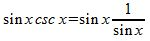To unlock this lesson you must be a Study.com Member.

Register to view this lesson

Are you a student or a teacher?